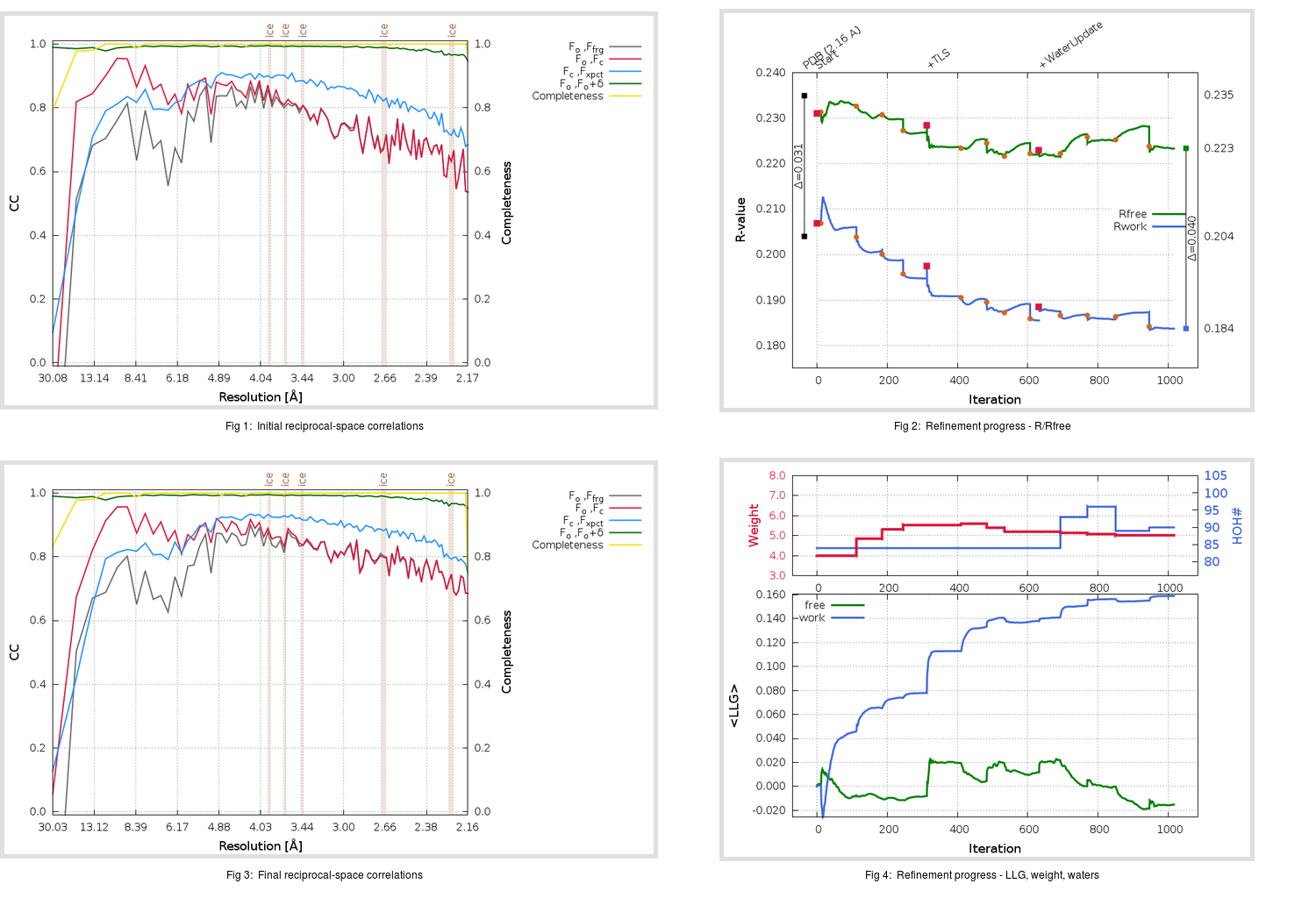Content:

```    Diffraction limits & principal axes of ellipsoid fitted to diffraction cut-off surface:
2.117         0.8950   0.0000   0.4461       a* + 0.020 c*
2.112         0.0000   1.0000   0.0000       b*
2.075        -0.4461   0.0000   0.8950      -0.645 a* + 0.764 c*
```

## Deposited

` `
 Date deposited Date data collection Resolution R, Rfree 20200126 20200112 2.16 0.2020 0.2350

Molprobity (CCP4 7.0 version) summary:

```Ramachandran outliers =   0.00 %
favored =  97.05 %
Rotamer outliers      =   0.38 %
C-beta deviations     =     0
Clashscore            =   5.03
RMS(bonds)            =   0.0060
RMS(angles)           =   0.97
MolProbity score      =   1.43
Resolution            =   2.16
R-work                =   0.2020
R-free                =   0.2350
```

```Number of waters      =    84

<B> (all atoms) =   42.82 ( sd =   11.47 ) for       2500 non-hydrogen atoms
<B>   (protein) =   42.68 ( sd =   11.53 ) for       2387 non-hydrogen atoms
<B>     (water) =   44.24 ( sd =    7.65 ) for         84 non-hydrogen atoms
<B>    (others) =   50.35 ( sd =   12.20 ) for         29 non-hydrogen atoms

B min/max       (all non-hydrogen atoms) =   16.91 /   89.99
B min/max   (protein non-hydrogen atoms) =   16.91 /   89.99
B min/max     (water non-hydrogen atoms) =   27.37 /   63.41
B min/max     (other non-hydrogen atoms) =   30.76 /   70.02
```

## BUSTER (re-)refinement

` `

Molprobity (CCP4 7.0 version) summary:

```Ramachandran outliers =   0.00 %
favored =  97.70 %
Rotamer outliers      =   3.02 %
C-beta deviations     =     0
Clashscore            =   2.93
RMS(bonds)            =   0.0119
RMS(angles)           =   1.53
MolProbity score      =   1.51
Resolution            =   2.16
R-work                =   0.1837
R-free                =   0.2233
```

```Number of waters      =    90

<B> (all atoms) =   44.23 ( sd =   12.60 ) for       2506 non-hydrogen atoms
<B>   (protein) =   43.92 ( sd =   12.56 ) for       2387 non-hydrogen atoms
<B>     (water) =   50.90 ( sd =   12.47 ) for         90 non-hydrogen atoms
<B>    (others) =   49.41 ( sd =    8.37 ) for         29 non-hydrogen atoms

B min/max       (all non-hydrogen atoms) =   22.23 /  101.07
B min/max   (protein non-hydrogen atoms) =   22.23 /  101.07
B min/max     (water non-hydrogen atoms) =   26.09 /   93.92
B min/max     (other non-hydrogen atoms) =   36.01 /   60.77
```

Refinement progression:Results:

` `
 File Remark 6LU7_aB_refine.01_03_refine.pdb.gz exact refinement commands are in header 6LU7_aB_refine.01_03_refine.mtz.gz including original deposited data and several re-refinement map coefficients 6LU7_aB_refine.01_03_BUSTER_model.cif.gz including any non-standard compound restraints 6LU7_aB_refine.01_03_BUSTER_refln.cif.gz